Documentation

plot

Plot timeseries

Description

example

plot(ts) plots the timeseries data in ts against time, interpolating values between samples.

plot(ts,specs) plots the timeseries data using a line graph and applies the specified specs to lines, markers, or both. You can also specify name-value pairs to define Line Properties.

Examples

collapse all

Create a time series object, set the start date, and then plot the time vector relative to the start date.

x = [2 5 8 2 11 3 6];
ts1 = timeseries(x,1:7);

ts1.Name = 'Daily Count';
ts1.TimeInfo.Units = 'days';
ts1.TimeInfo.StartDate = '01-Jan-2011';     % Set start date.
ts1.TimeInfo.Format = 'mmm dd, yy';       % Set format for display on x-axis.

ts1.Time = ts1.Time - ts1.Time(1);        % Express time relative to the start date.

plot(ts1)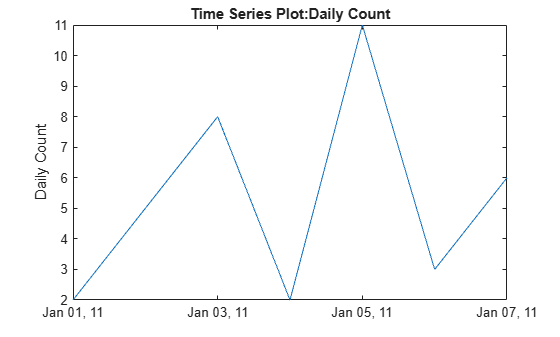Create two time series objects from traffic count data, and then plot them in sequence on the same axes. Add an event to one series, which is automatically displayed with a red marker.

count1 = timeseries(count(:,1),1:24);
count1.Name = 'Oak St. Traffic Count';
count1.TimeInfo.Units = 'hours';
plot(count1,':b')
grid on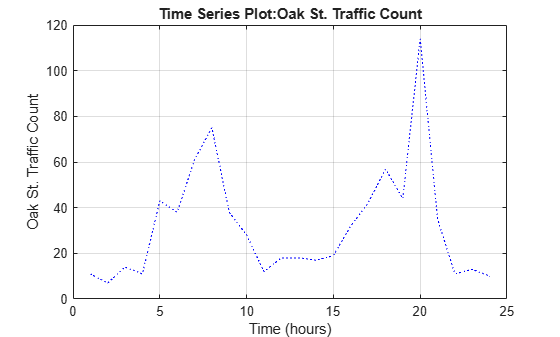Obtain time of maximum value and add it as an event:

[~,index] = max(count1.Data);
max_event = tsdata.event('peak',count1.Time(index));
max_event.Units = 'hours';

Add the event to the time series:

Replace plot with new one showing the event:

plot(count1,'.-b')
grid on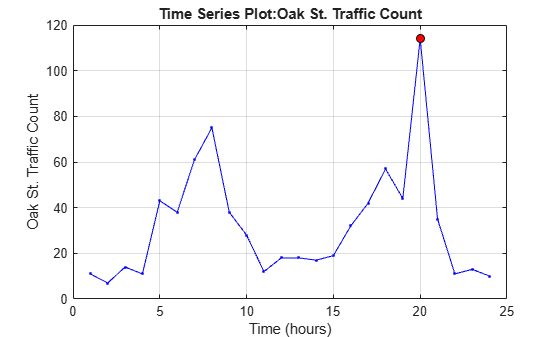Make a new time series object from column 2 of the same data source:

count2 = timeseries(count(:,2),1:24);
count2.Name = 'Maple St. Traffic Count';
count2.TimeInfo.Units = 'Hours';

Turn hold on to add the new data to the plot:

hold on

The plot method does not add labels to a held plot. Use property/value pairs to customize markers:

plot(count2,'s-m','MarkerSize',6),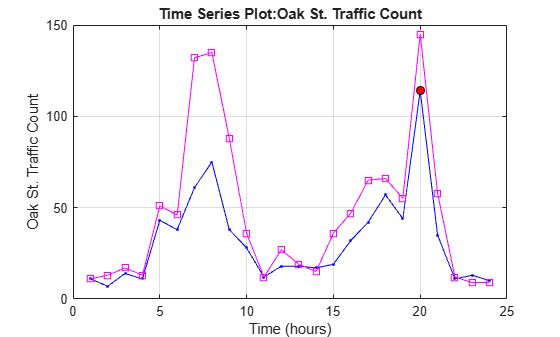Labels are erased, so generate them manually:

title('Time Series: Oak Street and Maple Street')
xlabel('Hour of day')
ylabel('Vehicle count')

Add a legend in the upper left:

legend('Oak St.','Maple St.','Location','northwest')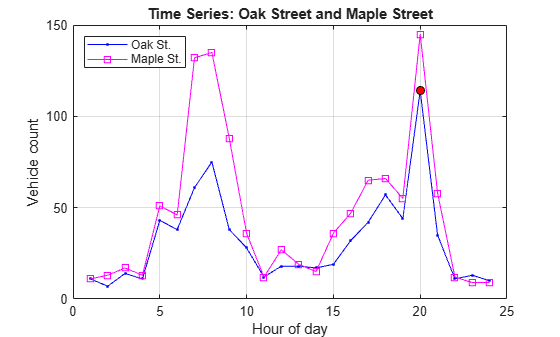Input Arguments

collapse all

Input timeseries, specified as a scalar.

Line specifications, specified as a character vector defining the appearance of lines, markers, or both. See LineSpec for more information. You can also specify name-value pairs to define Line Properties.

Data Types: char

Tips

• The plot function generates titles and axis labels automatically. These labels are:

• Plot Title — 'Time Series Plot: <name>'

where <name> is the string assigned to ts.Name, or by default, 'unnamed'

• X-Axis Label — 'Time (<units>)'

where <units> is the value of the ts.TimeInfo.Units field, which defaults to 'seconds'

• Y-Axis Label — '<name>'

where <name> is the string assigned to ts.Name, or by default, 'unnamed'

• You can place new time series data on a time series plot (by setting hold on, for example, and issuing another timeseries/plot command). When you add data to a plot, the title and axis labels become blank strings to avoid labeling confusion. You can add your own labels after plotting using the title, xlabel, and ylabel commands.

• Time series events, when defined, are marked in the plot with a circular marker with red fill. You can also specify markers for all data points using a linespec or name/value syntax in addition to any event markers your data defines. The event markers plot on top of the markers you define.

• The value assigned to ts.DataInfo.Interpolation.Name controls the type of interpolation the plot method uses when plotting and resampling time series data. Invoke the timeseries method setinterpmethod to change default linear interpolation to zero-order hold interpolation (staircase). This method creates a new timeseries object, with which you can overwrite the original one if you want. For example, to cause time series ts to use zero-order hold interpolation, type the following:

ts = ts.setinterpmethod('zoh');